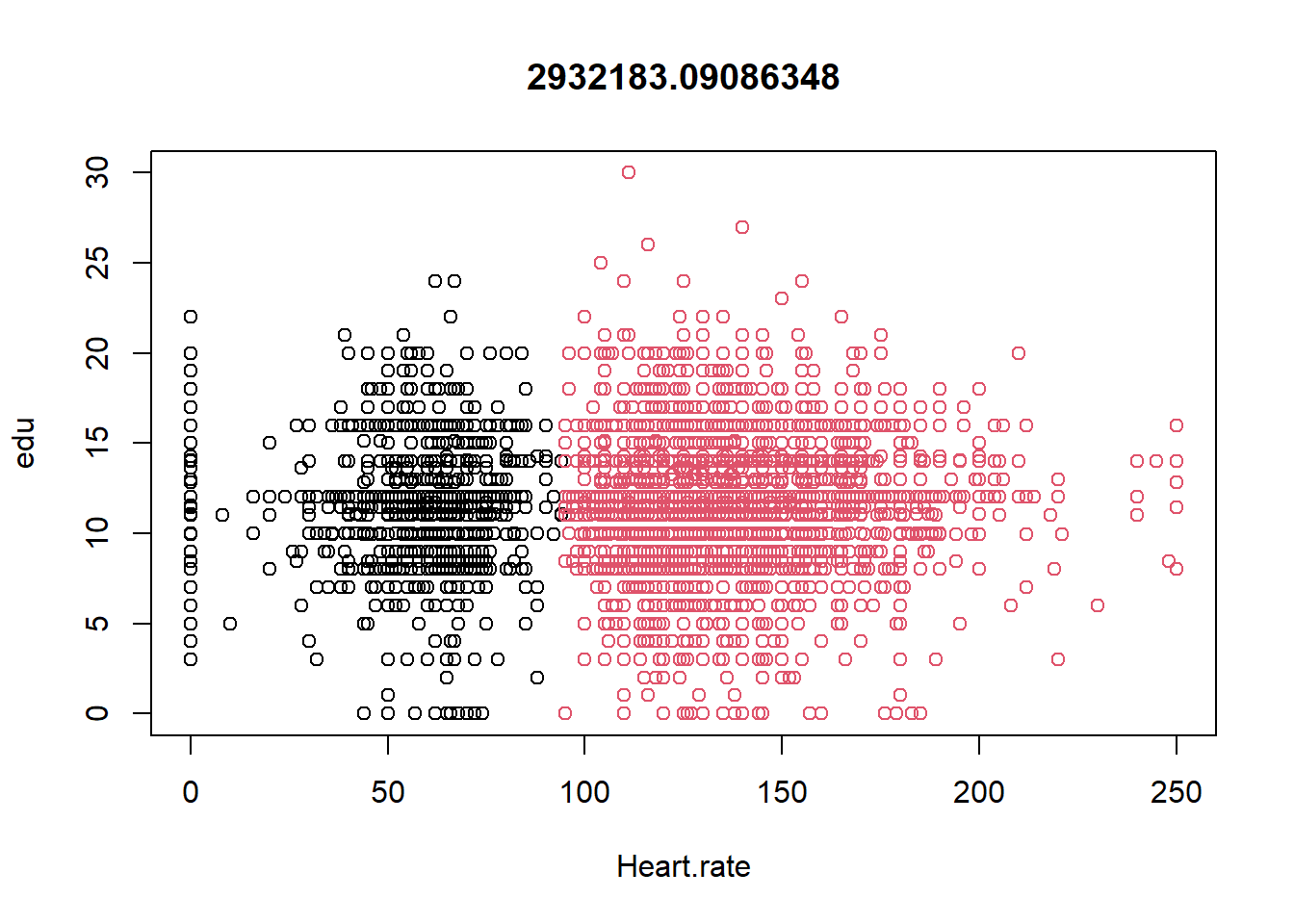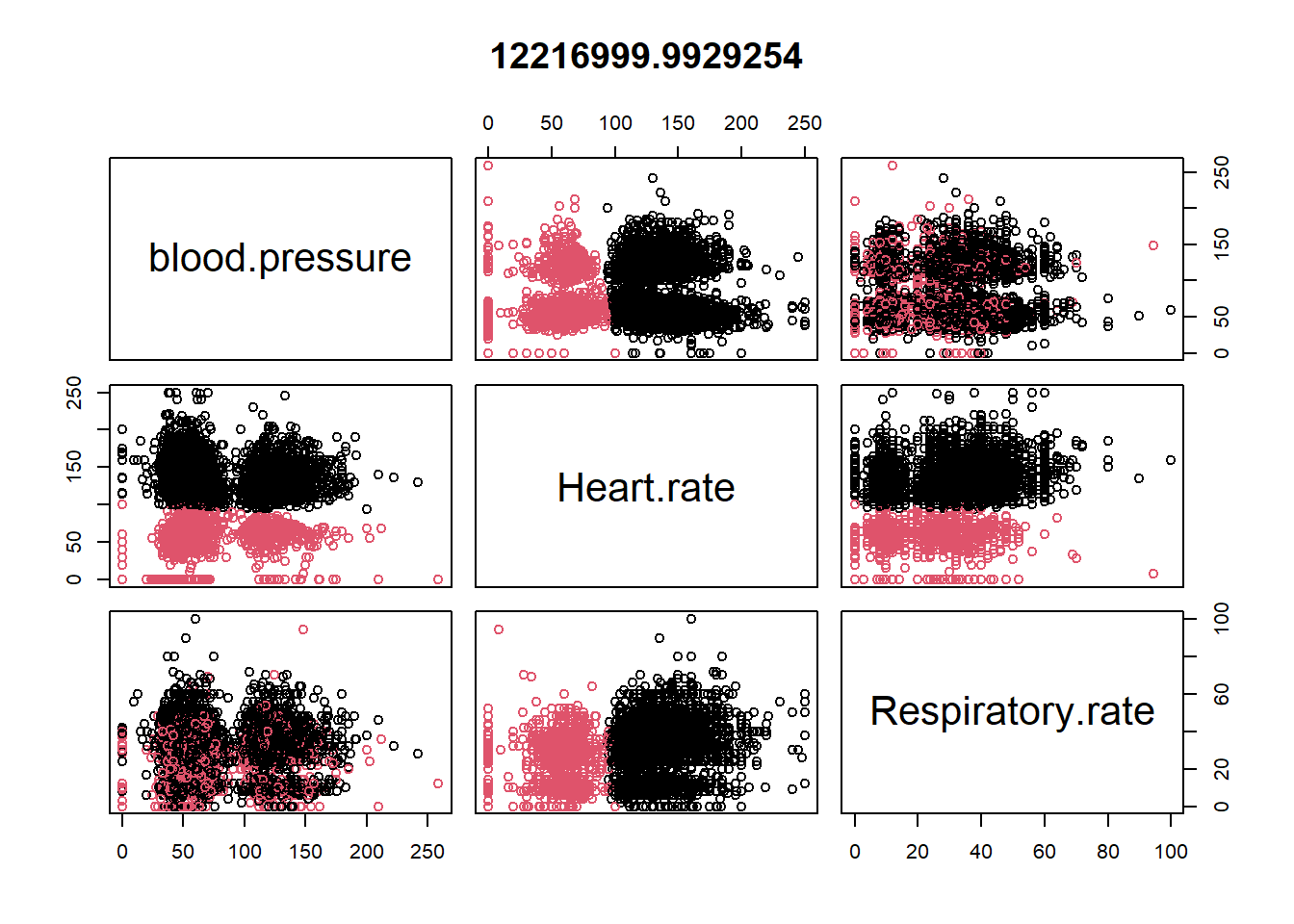# Chapter 7 Unsupervised Learning

Clustering is an unsupervised learning algorithm. These algorithms can classify data into multiple groups. Such classification is based on similarity.

Group characteristics include (to the extent that is possible)

• low inter-class similarity: observation from different clusters would be dissimilar
• high intra-class similarity: observation from the same cluster would be similar

Within-cluster variation will be thus minimized by optimizing within-cluster sum of squares of Euclidean distances (ref)## 7.1 K-means

K-means is a very popular clustering algorithm, that partitions the data into $$k$$ groups.

Algorithm:

• Determine a number $$k$$ (e.g., could be 3)
• randomly select $$k$$ subjects in a data. Use these points as staring points (centers or cluster mean) for each cluster.
• By Euclidean distance measure (from the initial centers), try to determine in which cluster the remaining points belong.
• compute new mean value for each cluster.
• based on this new mean, try to determine again in which cluster the data points belong.
• process continues until the data points do not change cluster membership.

## 7.2 Read previously saved data

ObsData <- readRDS(file = "data/rhcAnalytic.RDS")

### 7.2.1 Example 1

datax0 <- ObsData[c("Heart.rate", "edu")]
kres0 <- kmeans(datax0, centers = 2, nstart = 10)
kres0$centers ## Heart.rate edu ## 1 54.55138 11.44494 ## 2 134.96277 11.75466 plot(datax0, col = kres0$cluster, main = kres0$tot.withinss)### 7.2.2 Example 2 datax0 <- ObsData[c("blood.pressure", "Heart.rate", "Respiratory.rate")] kres0 <- kmeans(datax0, centers = 2, nstart = 10) kres0$centers
##   blood.pressure Heart.rate Respiratory.rate
## 1       80.10812  135.08956         29.85267
## 2       73.71684   54.95789         22.76723
plot(datax0, col = kres0$cluster, main = kres0$tot.withinss)### 7.2.3 Example with many variables

datax <- ObsData[c("edu", "blood.pressure", "Heart.rate",
"Respiratory.rate" , "Temperature",
"PH", "Weight", "Length.of.Stay")]
kres <- kmeans(datax, centers = 3)
#kres
head(kres$cluster) ##  1 1 1 3 1 2 kres$size
##  2793 1688 1254
kres$centers ## edu blood.pressure Heart.rate Respiratory.rate Temperature PH ## 1 11.85833 54.26924 136.37451 29.76119 37.85078 7.385249 ## 2 11.54214 128.33886 126.12026 29.36611 37.68129 7.401027 ## 3 11.46134 65.47249 53.24242 22.65973 37.01597 7.378482 ## Weight Length.of.Stay ## 1 68.63384 23.42356 ## 2 66.68351 20.68128 ## 3 67.57291 18.58931 aggregate(datax, by = list(cluster = kres$cluster), mean)
##   cluster      edu blood.pressure Heart.rate Respiratory.rate Temperature
## 1       1 11.85833       54.26924  136.37451         29.76119    37.85078
## 2       2 11.54214      128.33886  126.12026         29.36611    37.68129
## 3       3 11.46134       65.47249   53.24242         22.65973    37.01597
##         PH   Weight Length.of.Stay
## 1 7.385249 68.63384       23.42356
## 2 7.401027 66.68351       20.68128
## 3 7.378482 67.57291       18.58931
aggregate(datax, by = list(cluster = kres\$cluster), sd)
##   cluster      edu blood.pressure Heart.rate Respiratory.rate Temperature
## 1       1 3.162485       11.93763   23.13140         13.67791    1.781692
## 2       2 3.091605       18.58070   27.68369         14.08169    1.610746
## 3       3 3.160538       31.89150   23.63993         13.60831    1.832389
##          PH   Weight Length.of.Stay
## 1 0.1082140 27.99506       29.01143
## 2 0.1009567 32.15078       23.37223
## 3 0.1226041 26.87075       20.82024

## 7.3 Optimal number of clusters

require(factoextra)
fviz_nbclust(datax, kmeans, method = "wss")+
geom_vline(xintercept=3,linetype=3)Here the vertical line is chosen based on elbow method (ref).

## 7.4 Discussion

• We need to supply a number, $$k$$: but we can test different $$k$$s to identify optimal value
• Clustering can be influenced by outliners, so median based clustering is possible
• mere ordering can influence clustering, hence we should choose different initial means (e.g., nstart should be greater than 1).